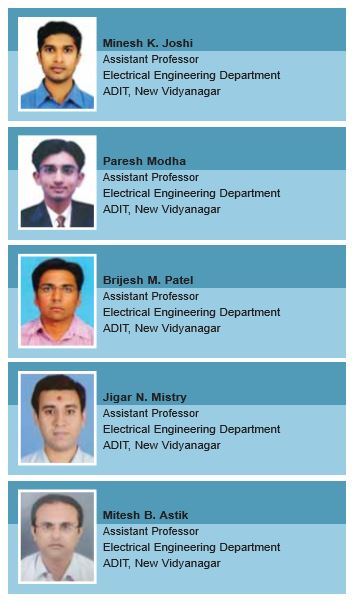# Managing 12 Pulse Converters

This article discusses 12 Pulse Converter Circuitry commonly found in Variable Speed Drives. The simulation of 12 pulse converters performs in both the Balanced and Unbalanced condition of Line voltages as well as frequency... - Minesh K. Joshi, Paresh Modha, Paresh Modha, Jigar N. Mistry, Mitesh B. Astik

Rectifier is a power electronic circuit that is used to convert AC signals into DC signals. A rectifier is a ‘power processor that should give a DC output voltage with a minimum amount of harmonic contents.’

We know that a standard six pulse rectifier caused a predictable harmonic spectrum consisting of the 5th, 7th, 11th, 13th, 17th, 19th, 23th…harmonics. For three phase power system rectifiers, the harmonics, which will normally be present in the input current harmonic spectrum, can be identified by the following equation: h = NP±1 Where, N is an integer (1, 2,3,4,5…) and P is the number of rectifier pulses on the DC bus waveform for one cycle of a input voltage. In this article, we deal with the reduction of Total Harmonic Distortion using Multi-pulse AC to Conversion scheme.

An increasing number of pulses reduce the harmonic contents in a line current. However, this technique is profound, has high cost, complex construction, and needs to be large in size.

SIMULATION OF 12 PULSE CONVERTERSFigure 1: Simulation Model of 12 Pulse Converters…

Since two valves conduct at the same time in each bridge, there will be 4 valves conducting at a time in a 12-pulse bridge converter.

MATHEMATICAL MODELING OF BRIDGE CONVERTER

The AC Voltage of the lower bridge lags that of the upper bridge by 30° (electrical).
For Upper Bridge we have:The Lower Bridge having 30° phase shift, the output voltage is:The Output Voltage,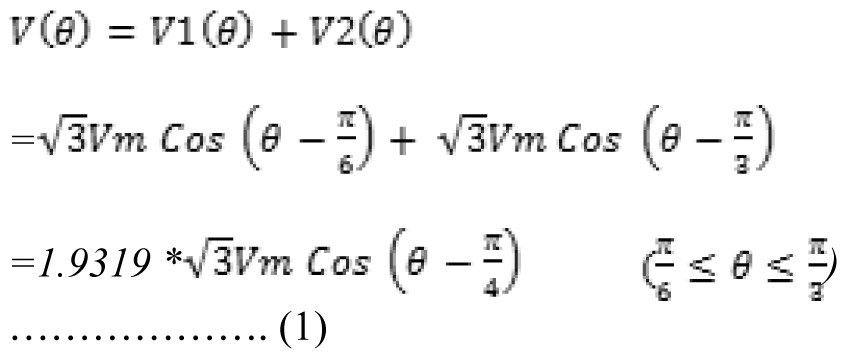The Average Value of V (Θ) for the period Π/6 to Π/3 is:The peak to peak ripple is:In terms of DC Voltage:
PPR = 0.114 x 0.3026 V
= 0.0345……………… (3)
Thus, it can be seen that the ripple is 3.45% in DC voltage.

TEST SYSTEM PARAMETERSSIMULATIONS RESULTS AND DISCUSSIONS

The model of 12 pulse converter is simulated for different value of load. The comparison between the Input line current when the load is 30 percent and full loads it is shown in the figure. Moreover, the simulation is performed for different value of line voltage under balanced and unbalanced conditions as well as with the balanced and unbalanced conditions with the frequency also. In this article, the following case will be discussed. As per the common practical tolerance limit by standard the fluctuation in voltage is assume up to ±5% and in frequency is up to ±3%.

1. Balanced 3-phase line voltages Va=Vb=Vc=230V, Frequency are the same in all phases=50Hz
2. Unbalanced with Va = 230V +5%, while the other two voltages are set equal to 230V
3. Unbalanced with both Va and Vb =230V+5%, while Vc =230V
4. Unbalanced with both Va =230V+5%, Vb =230V, and Vc =230-5%V
5. Unbalanced with both Va =230V-5%, Vb =230-5%V, and Vc =230V
6. Balanced Line Voltages Va = Vb = Vc =230V, fa =50+3%, fb =50-3%, fc =50
7. Balanced Line Voltages Va = Vb = Vc =230V, fa =50+3%, fb = fc =50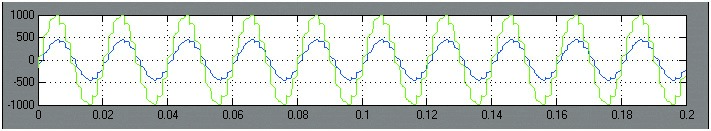Figure 2: Comparison of input line currents at 30% load & at full load…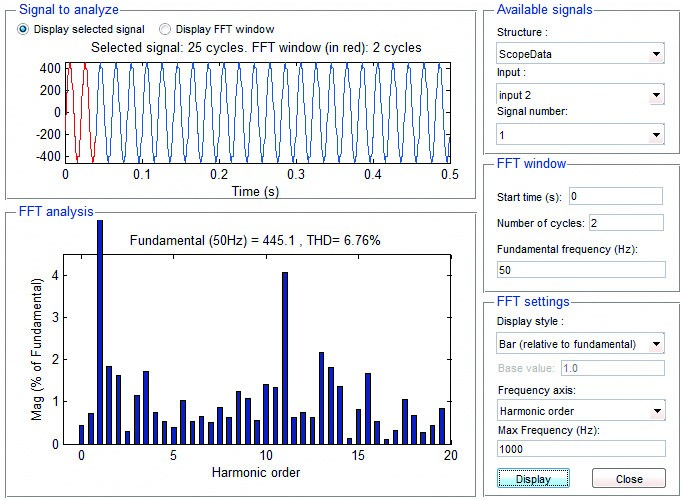Figure 3: THD Analysis in input line current for balanced system… V line=230V, f=50HzFigure 3: Comparison of %THD in input line current in Case 1, 2 and 3…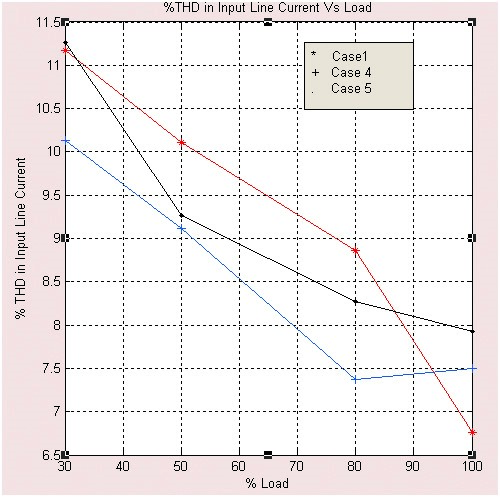Figure 4: Comparison of %THD in input line current
in Case 1, 4 and 5…

As we can see the graph of input Line current %THD and % Load of the figure 3 & 4, where the comparison is done of case 1,2,3 and case 1,4,5 in the full load condition, where all the line voltages and frequency are in balanced condition produces the least amount of harmonics. Whereas the interesting phenomenon to note down is that when load is  from 30% to 80%, the unbalanced condition in line in voltages produces least amount of harmonics then the balanced condition.Figure 5: Comparison of %THD in input line current
in Case 1, 6 and 7…

As we can see the graph of input Line current THD and percentage Load of the figure 2, where the comparison is done of case 1, case 6 and case 7. When the line voltages are in balanced condition but the frequency is in unbalanced then except the full load condition the unbalanced condition produces the least amount of harmonics when the load is in between 30% to 80%.

OBSERVATION TABLECONCLUSION

Modeling and Simulation of twelve pulse bridge converter are discussed in this article. With simulation results, engineers will be able to predict beforehand the level of input current harmonics, dealing with practically in three phase system.

In short, to exhibit the validity of the model, here different seven cases examples are simulated and how the input line current THD are affected under balanced and unbalanced condition with variation of load is deeply discussed.

Moreover, the model discussed here is based on ideal components; the user may modify the model by adding some filtering technique to reduce the THD in input line current for future pursuits.